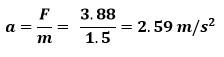## Incline Planes

Learn the forces involved in incline planes with and without friction. See how to solve for acceleration of an object created by the net force.

Forces on an incline

• Fw: Weight
• Longest Vector Straight Down

Every other force is the result of the weight

• F: Perpendicular Force
• Force pushing into the hill
• F: Parallel Force
• Force pushing down the hill
• FN : Normal Force
• Reaction to the force pushing into the hill (F)and equal and opposite to it
• Ff : Force of Friction
• Reaction to the force pushing down the hill (F)
• Ө : Angle of Incline
• Same as angle in the right triangle### Incline Planes and Trig Functions

Depending what the incline plane question is asking you will use the SOH CAH TOA trig functions.  The most common case will be when you are given a mass on a hill and provided the hills incline(Ө).  Start by finding weight (FW = mg (g = 9.8 m/s2).  Because you have weight and an incline angle, you now can find other forces involved.  These are the most common rearranged forms.

• F= (cosӨ)(hyp)
• F= (sinӨ)(hyp)
• FN = F= (cosӨ)(hyp)

Depending on how a the questions is asked you may need any of the trig functions including tangent.### Example Problems (Incline Plane)

1) How much a 1.5 kg block accelerate down a 15° frictionless incline plane?

Start by drawing out the incline and all the forces involvedNext find the weight of the 1.5 kg mass.

Fw = mg
Fw = (1.5)(10) = 15N down

Because weight causes the other forces it is the longest vector which is always directed straight down

Now find the other important forces involved

Since this problem is frictionless parallel force will be our net force.F⸗ = opp = (sinӨ)(Fw)
= (sin15)(15)
= 3.88 N

Use net force to determine acceleration

F = maThe acceleration is directed downhill.2) How much would the block accelerate if it was wood and the incline was wood and there was friction?

F⸗ = 3.88 N was found in the previous problem

Find normal force needed for the friction equation

We need to find the force into the hill so we have the normal force pushing back perpendicular to the hill.  This will be used in the friction equation.F = (cos 15)(15) = 14.49 N

Now that we have the perpendicular force into the hill we know the normal force with the same magnitude.

FN = F = 14.49 N

Next do a check to see if the object will be moving in the first place (static friction)

Lets make sure is will be moving using (static friction) before we find out how much it will accelerate.  Below we used the coefficient of static friction (0.25) found in the diagram in the force of friction equation.

Ff = µFN

Ff = (0.25)(14.49) = 3.62 N

There is motion (acceleration) because 3.88 N of force downhill is greater that 3.62 N of static friction uphill.

Now that we know it moves find the kinetic frictional force

Ff = (0.20)(14.49) = 2.90 N

Determine the net force next which causes accelerationFnet = 3.88 – 2.90 = 0.98 N downhill

Solve for acceleration using the Fnet = ma equation.Since the net force was downhill the direction of acceleration caused by it is downhill.

3) How fast would the block be going after 3 seconds with friction?

Since nothing was stated about initial motion it started from rest.

vf = ?

vi = 0 m/s

a = 0.65 m/s2

t = 3s

Pick the right motion equation with these givens.vf = vi + at

vf = 0 + (0.65)(3) = 1.95 m/s downhill

Since the acceleration caused by net force was downhill the complete answer would also have the direction downhill.

Incline Plane Quiz

1 / 13Which letter above represents weight?

2 / 13Which letter above represents the parallel force?

3 / 13Which letter above represents normal force?

4 / 13Which letter above represents friction?

5 / 13Which number above is where the incline angle would be placed?

6 / 13

What happens to the normal force as the angle of incline increases?

7 / 13

What happens to the downhill force as the angle of incline increases?

8 / 13

What happens to the frictional force as the angle of incline increases?

9 / 13

What is the weight of a 4.0 kg object on a 25° frictionless Incline Plane? (Use g = 9.8 m/s2)

10 / 13

What is the acceleration of a moving object on a 4.0 kg object on a 25° Incline Plane that has a coefficient of friction with the object of 0.43?

11 / 13

What is the Normal force on a 4.0 kg object on a 25° Incline Plane?

12 / 13

What is the downhill force on a 4.0 kg object on a 25° Incline Plane?

13 / 13

What is the frictional force of a moving object on a 4.0 kg object on a 25° frictionless Incline Plane that has a coefficient of friction with the object of 0.43?